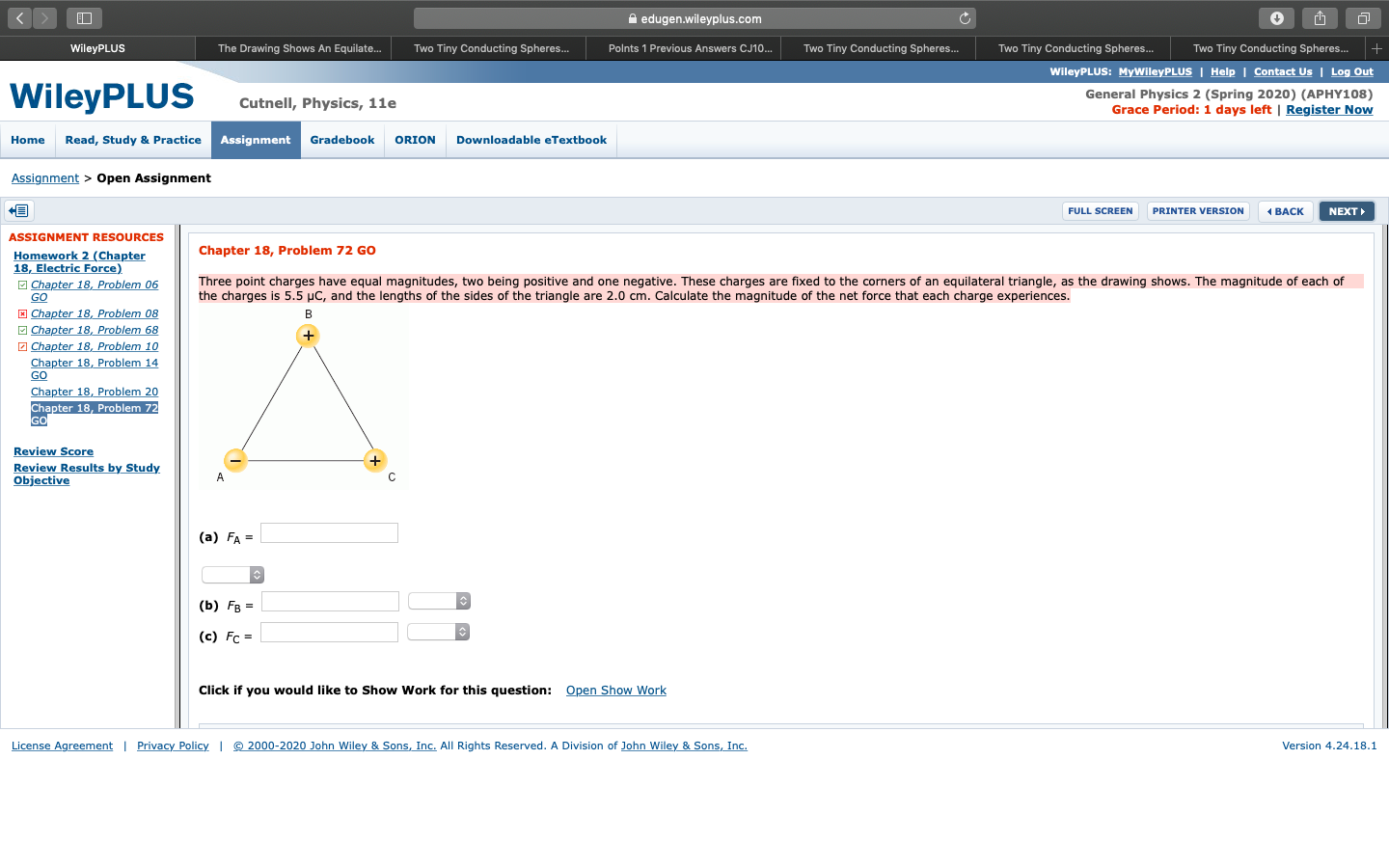Home / Answered Questions / Other / three-point-charges-have-equal-magnitudes-two-being-positive-and-one-negative-these-charges-are-fixe-aw588

# (Solved): Three Point Charges Have Equal Magnitudes, Two Being Positive And One Negative. These Charges Are Fi...

Three point charges have equal magnitudes, two being positive and one negative. These charges are fixed to the corners of an equilateral triangle, as the drawing shows. The magnitude of each of the charges is 5.5 Î¼C, and the lengths of the sides of the triangle are 2.0 cm. Calculate the magnitude of the net force that each charge experiences.